# 【数据结构与算法】Manacher算法详解

## Manacher算法

### 1. 引入

Manacher算法和KMP算法都是解决字符串相关题目的常见算法原型，但是各自解决的问题却不一样。

Manacher算法一开始是专门用来解决 "字符串中最长回文子串问题" 的，实际上Manacher算法中有一个非常重要的信息，使用它可以解决很多其他问题，这个信息就是：每一个位置的最长回文半径

### 4. 经典解法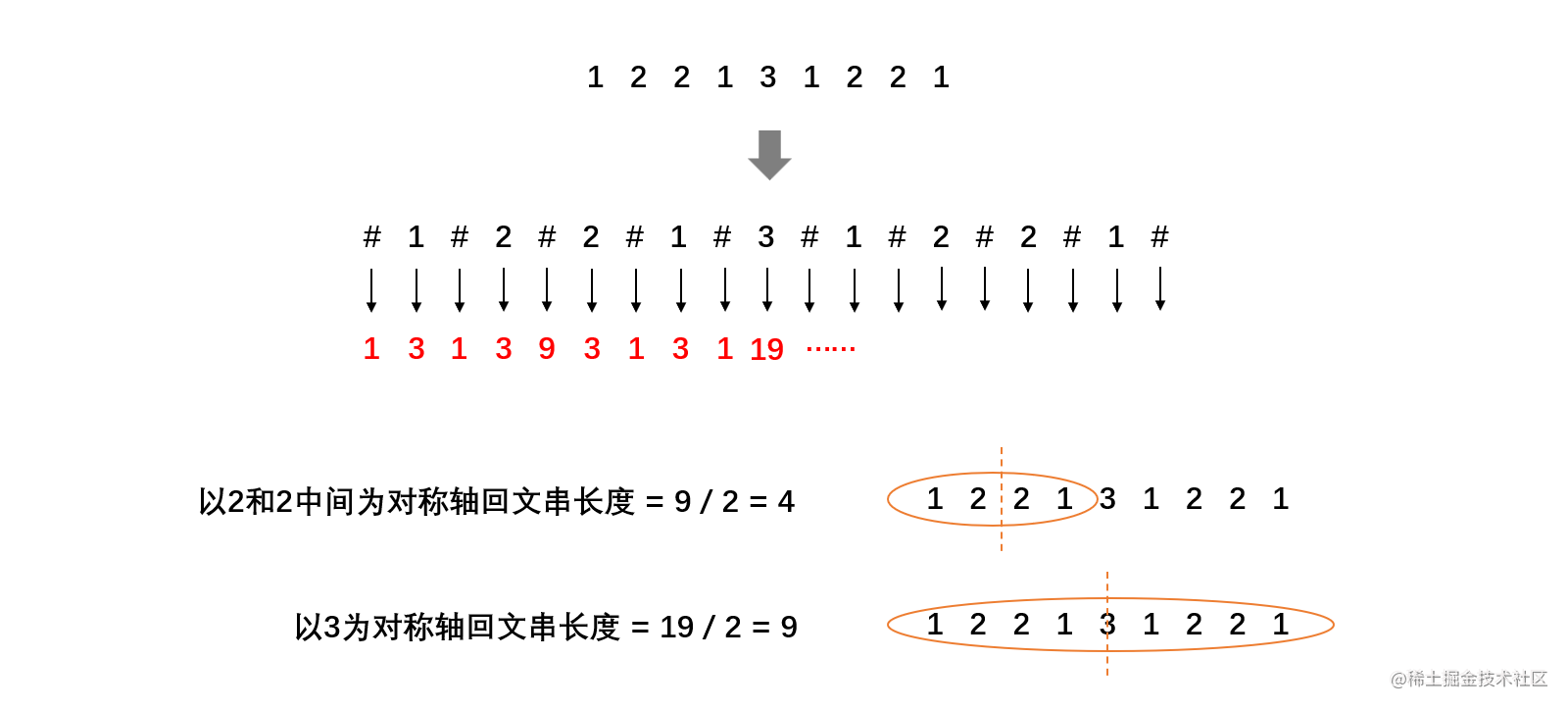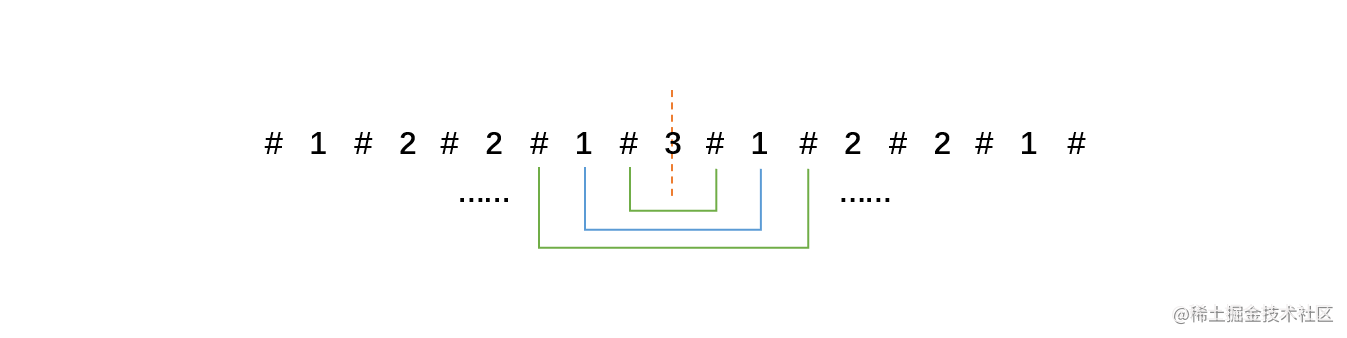``````public static int plalindrome(String str) {
if (str == null || str.length() == 0) {
return -1;
}

char[] c = str.toCharArray();

char[] newC = new char[c.length * 2 + 1];
newC = '#';

// c的指针
int i = 0;
// newC的指针
int j = 1;

// 使用辅助字符#处理原字符串
while (i < c.length && j < newC.length - 1) {
newC[j ++] = c[i ++];
newC[j ++] = '#';
}

return process(newC);
}

public static int process(char[] str) {
// 回文区域数组
int[] next = new int[str.length];

//构建回文区域数组
for (int i = 0; i < str.length; i ++) {
int j = i - 1;
int k = i + 1;
// 回文区域最少是1，为本身
int count = 1;
// 向两边每个字符进行匹配
while (j >= 0 && k != str.length) {
if (str[j] != str[k]) {
break;
}
j --;
k ++;
count = count + 2;
}
next[i] = count;
}

// 回文区域数组升序排序
Arrays.sort(next);

// 回文区域数组中最大的元素除以2就是最大回文子串长度
return next[next.length - 1] / 2;
}

### 5. 回文半径和回文直径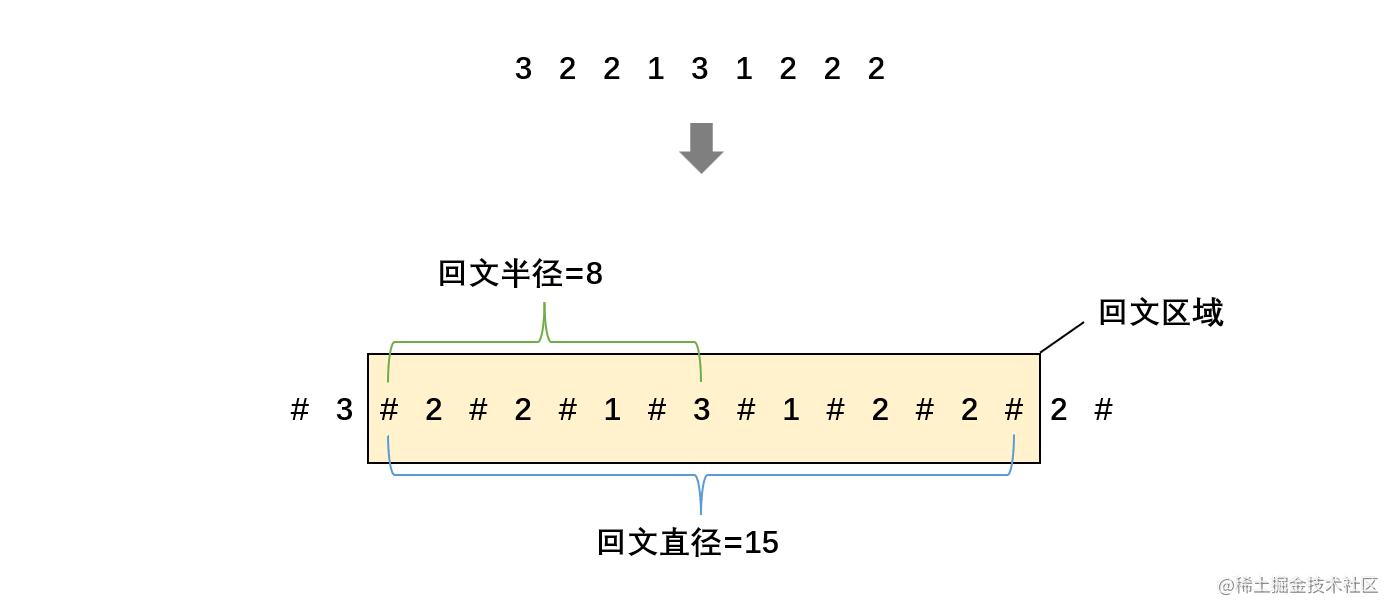### 6. Manacher算法

Manacher和经典解法的处理流程实际上是一样的，Manacher和KMP一样主要是设计了加速操作，Manacher能够将时间复杂度优化到O(N)。

Manacher算法设计中有三个重要点：

• 需要给被辅助字符处理的字符串中的每一个字符计算回文半径，从而构建一个回文半径数组。
• 设置一个变量 R，记录之前匹配回文区域的所有字符中，回文边界达到的最右下标（初值为-1）。
• 设置一个变量 C，和 R 一起用，记录当前取得最右下标的回文区域的中心点的下标（初值为-1，如果最右下标重合，按照原中心点的下标）。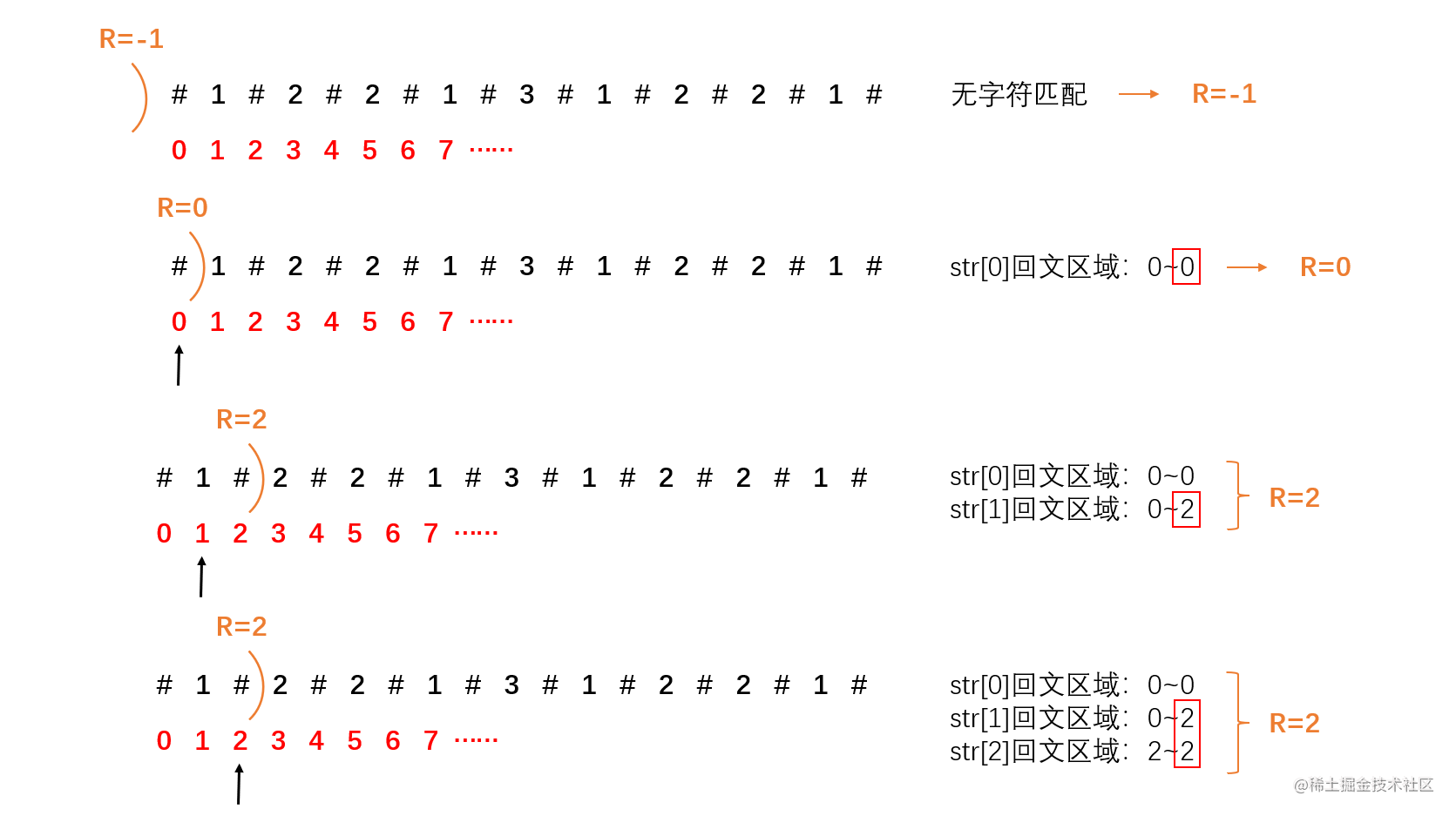R 永远是只增不减的，只要有字符的回文更靠右，下标就会更新给 R。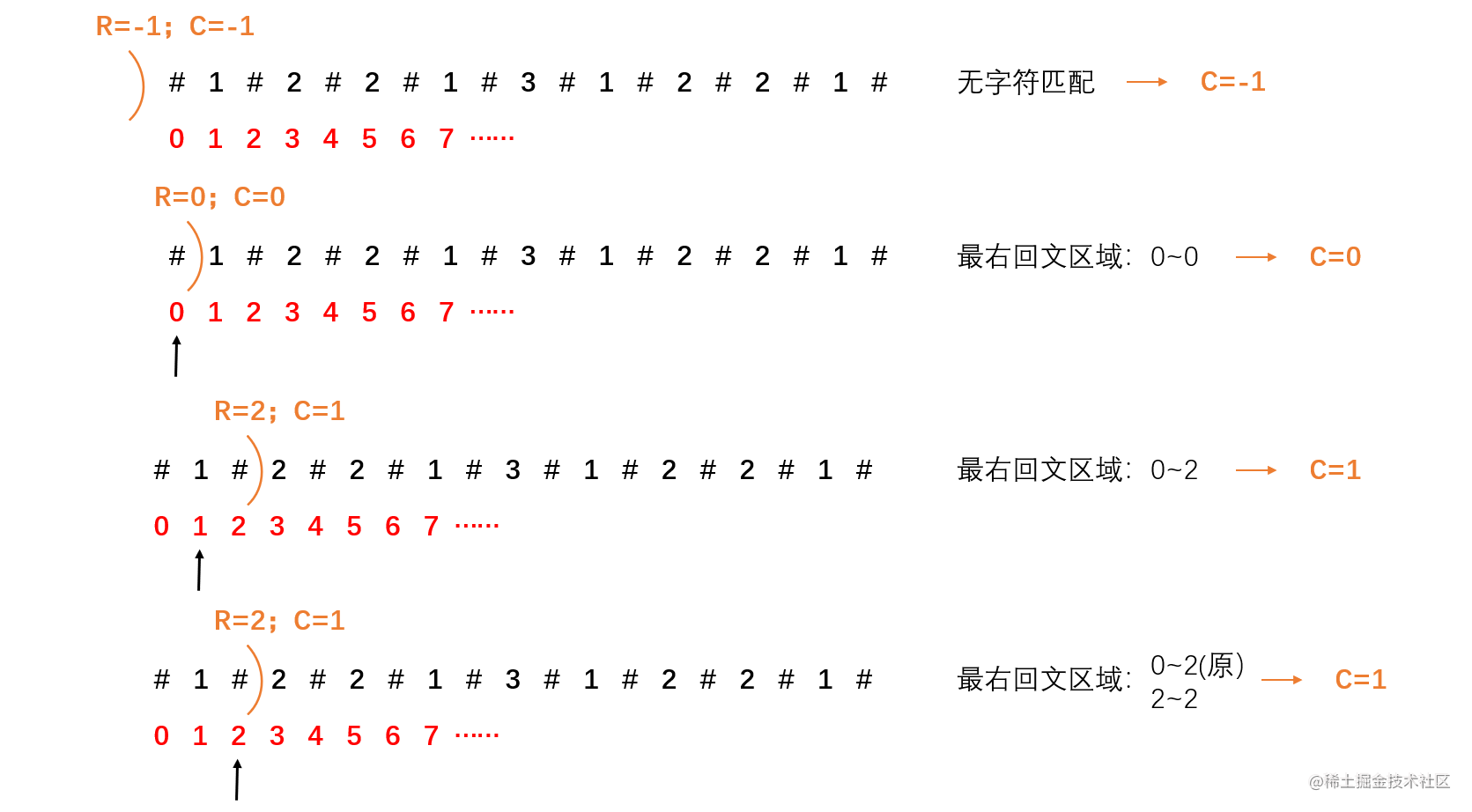C 也是永远只增不减的，R 更新 C 一定更新，R 不更新 C 一定不更新。

### 7. 流程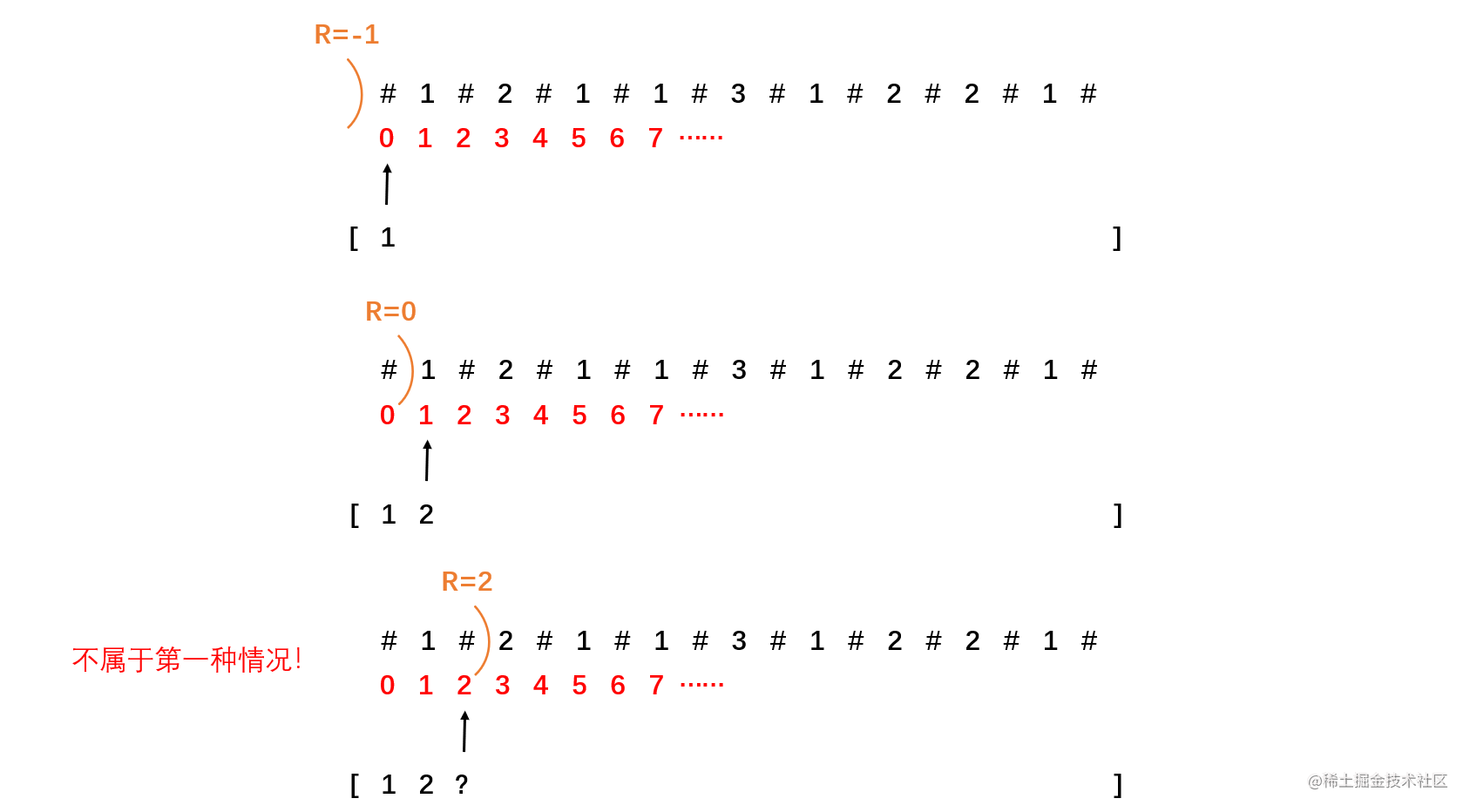i 为当前匹配的字符的位置，i‘ 是以 C 为对称轴所作的 i 的对称点。C、L 和R 一定都存在。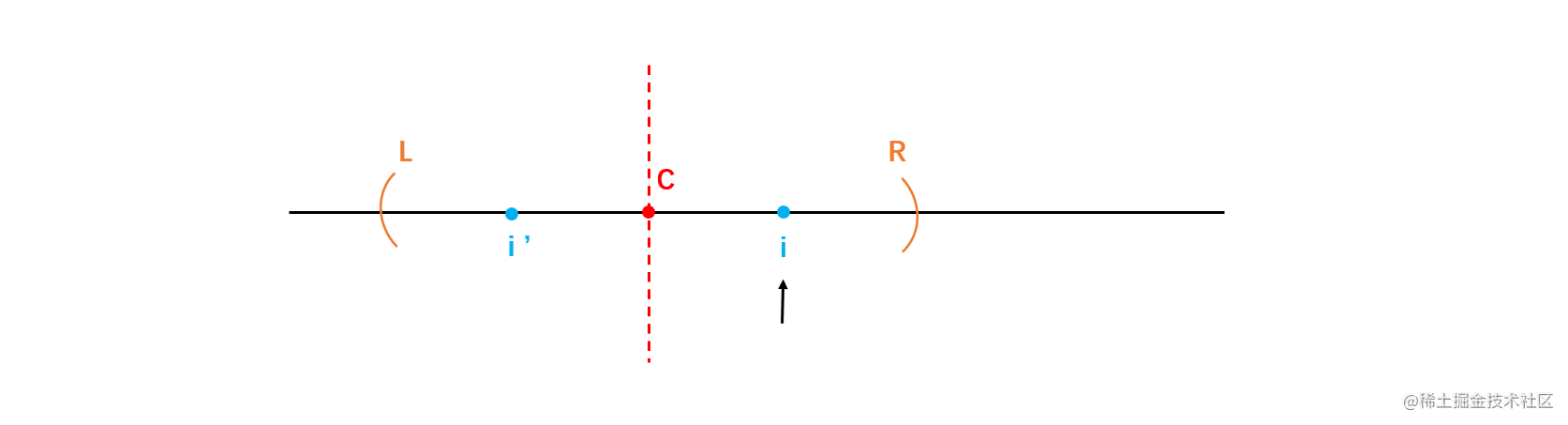i 和 C 是不可能重合的，因为 C 表示的是 i 之前字符构建的最长回文子串的中心点。当遍历到 i 位置时，C 一定已经遍历过了。

（1）第一种情况：i‘ 的回文区域完全在L~R的内部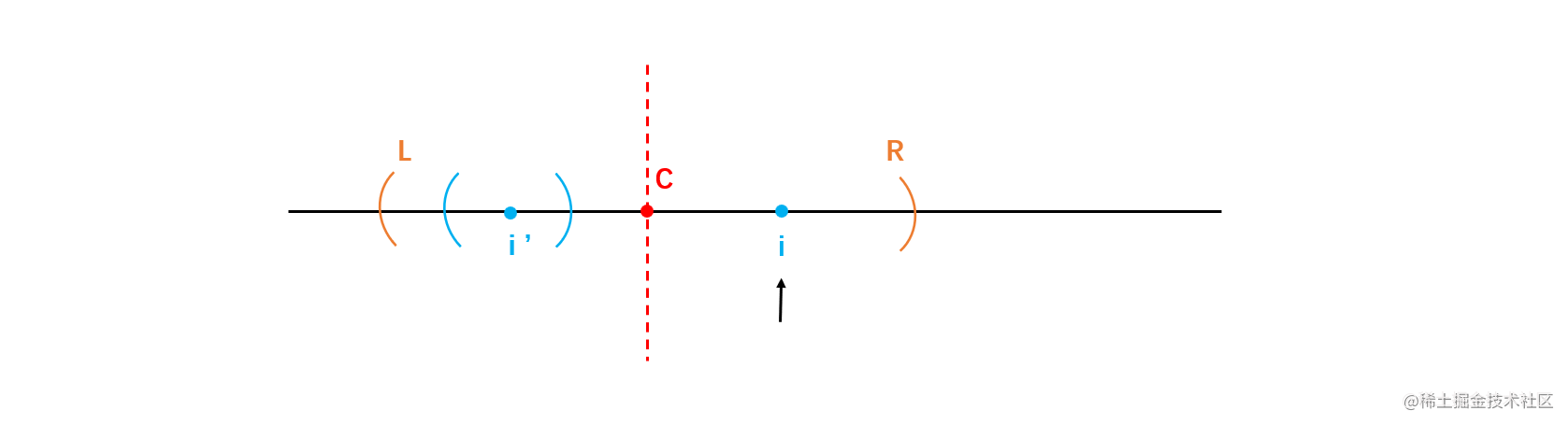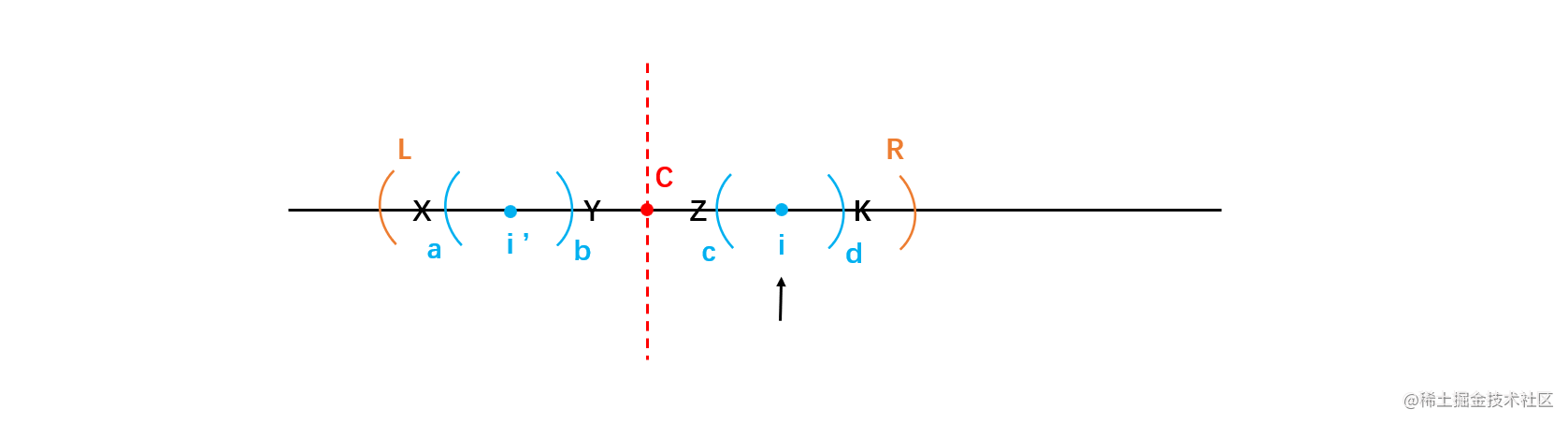（2）第二种情况：i‘ 的回文区域有一部分在 L~R 的外部。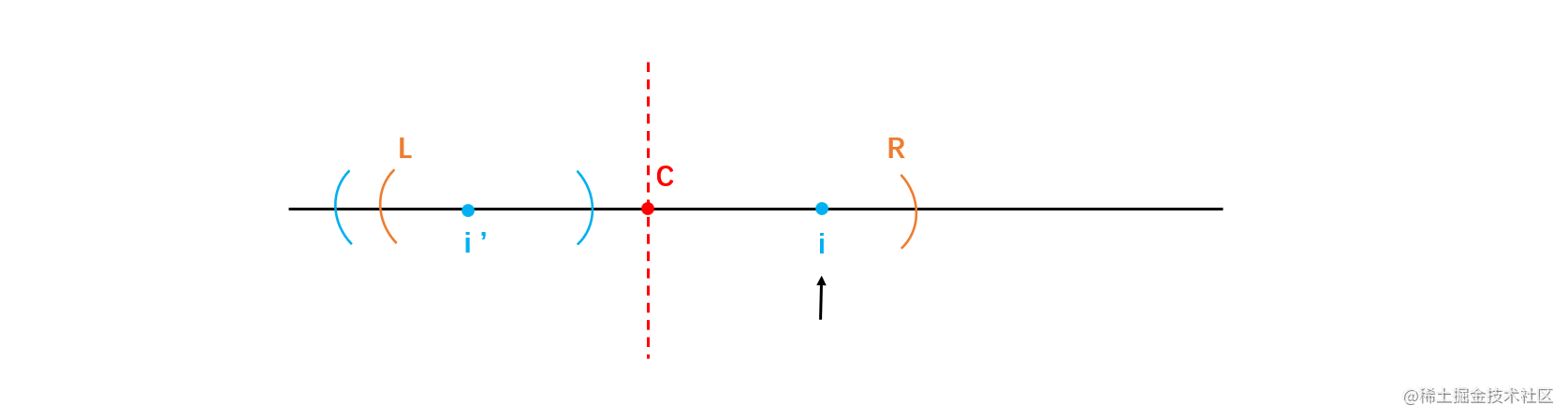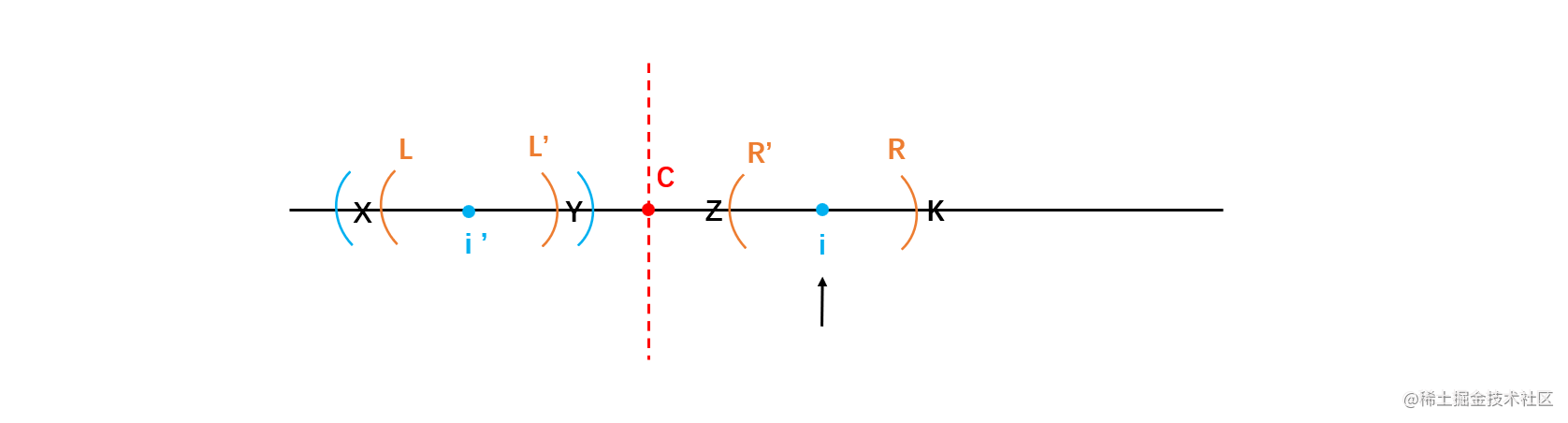L 作 i’ 的对称点 L'，R 作 i 的对称点 R'。

L~L‘ 和 R~R’ 一定是逆序关系，L~L‘ 是回文，因为 L~L’ 在 L~R的内部，因此 R~R’ 一定也是回文，因此 i 的回文区域至少与 L~L’ 等规模。为什么 R~R’ 不能更大？需要证明。

（3）第三种情况：i‘ 的回文区域的左边界正好和 L 重合。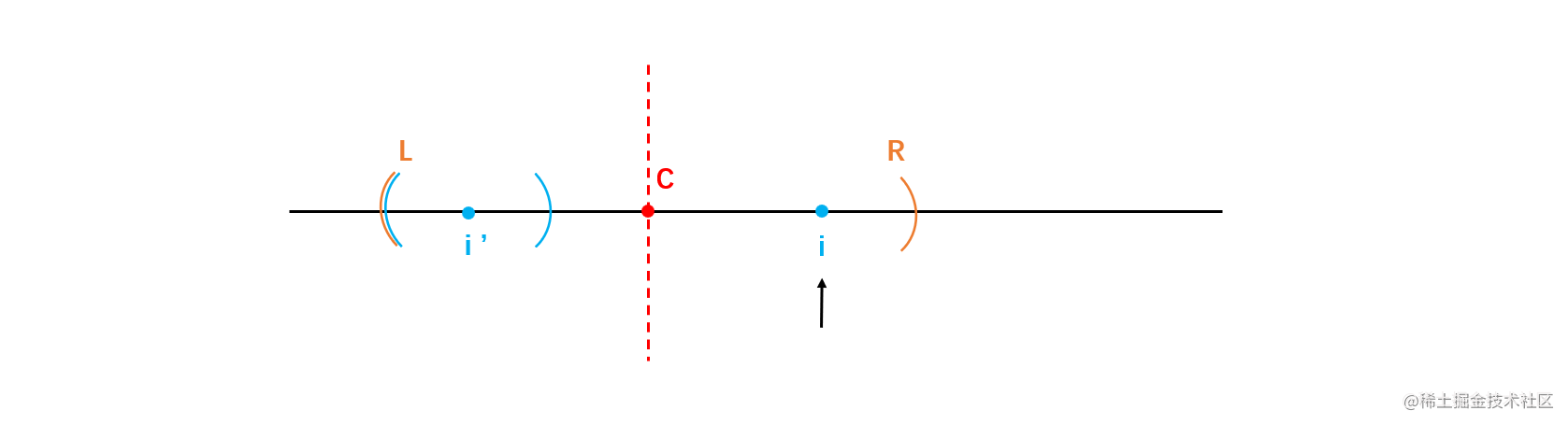### 8. 实现

``````public static int plalindrome(String str) {
if (str == null || str.length() == 0) {
return -1;
}

char[] c = str.toCharArray();

char[] newC = new char[c.length * 2 + 1];
newC = '#';

// c的指针
int i = 0;
// newC的指针
int j = 1;

// 使用辅助字符#处理原字符串
while (i < c.length && j < newC.length - 1) {
newC[j ++] = c[i ++];
newC[j ++] = '#';
}

return process(newC);
}

public static int process(char[] str) {
// 这里的R和定义中有些不同，这里是指i之前字符的回文区域最右边的下标的后一位，也就是说R-1才是回文区域最右边的下标
int R = -1;
// 中心
int C = -1;

// 回文半径数组
int[] next = new int[str.length];

for (int i = 0; i < str.length; i ++) {
// 先确定所有情况中最低回文半径
// 如果i > R，表示第一种大情况，最低回文半径为自身为1
// 如果 i < R，表示第二种大情况：
// 如果是第①种小情况，i'的回文半径会比R-i小，直接可以确定为i'的回文半径
// 如果是第②种小情况，i’的回文半径会比R-i大，直接可以确定为R-i
// 如果是第③种小情况，i’的回文半径和R-i相等，虽然不能确定最终的回文半径，但是可以确定最少就是i'的回文半径或R-i
next[i] = i < R ? Math.min(next[2 * C - i], R - i) : 1;

// 无论是遇到哪一种情况都会尝试着往外扩，因为只有第一种大情况和第二种大情况的第③种小情况需要向外扩，因此如果是第二中大情况的
// 第①种小情况或者第②种小情况虽然也会走向外扩的流程，但是第一次就会失败，从而跳出循环。
// 这是为了省略多个if-else所做的一个统一的流程，优化了代码的长度，并不会影响时间复杂度
while (i + next[i] < str.length && i - next[i] >= 0) {
if (str[i + next[i]] == str[i - next[i]]) {
next[i] ++;
} else {
break;
}
}

// 判断R和C是否需要更新
if (i + next[i] > R) {
// R向右扩
R = i + next[i];
// 此时i就是当前所有字符的回文区域达到最右的区域的中心点
C = i;
}
}

// 给next数组排序，找到最大的回文半径
Arrays.sort(next);
int maxPalindromeRadius = next[str.length - 1];

// 处理串中i位的回文半径长度 - 1 = 原串中以i为对称轴的回文子串长度
}

### 9. 时间复杂度

``````public static int process(char[] str) {
int R = ?;
int C = ?;

int[] next = new int[str.length];

for (int i = 0; i < str.length; i ++) {
if (i在R的外面) {
从i开始向外扩;
} else { // i在R的里面
if (i'的回文区域完全在L~R中) {
// O(1)的操作
返回i'的回文半径;
} else if (i'的回文区域的左边界在L的左边) {
// O(1)的操作
返回R-i;
} else { // i'的回文区域的左边界与L重合
从R开始向外扩;
}
}

排序获得最长的回文半径处理成原文的回文串长度返回
}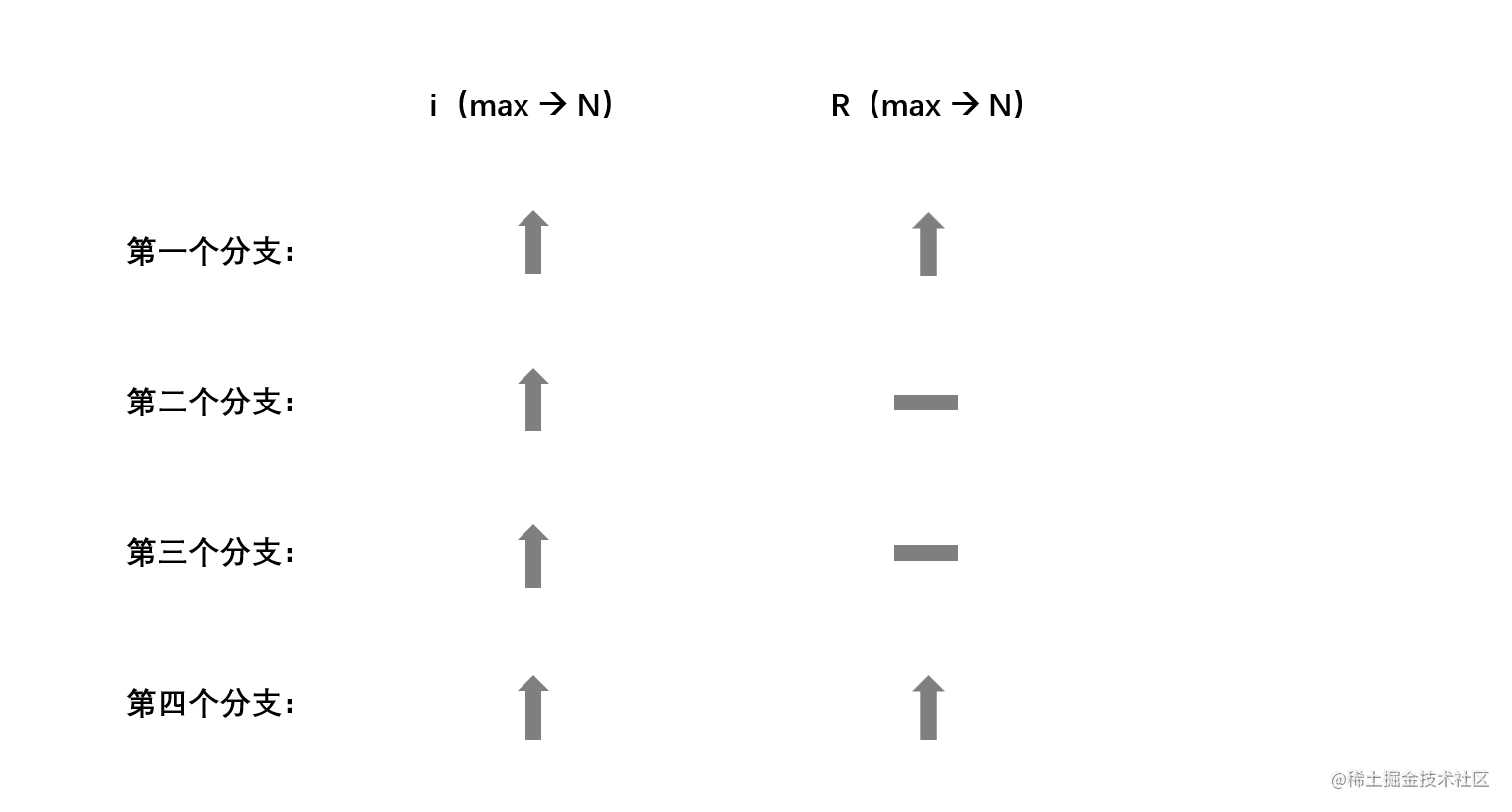i 只增不减，R 也是只增不减。

### 10. 引申

Manacher算法可以解决最大回文子串的问题，但远远不仅于此，通过回文半径数组next的信息可以解决非常多的回文问题。